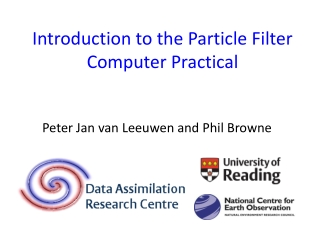DownloadDownload PresentationIntroduction to the Particle Filter Computer Practical

# Introduction to the Particle Filter Computer Practical

Download Presentation## Introduction to the Particle Filter Computer Practical

- - - - - - - - - - - - - - - - - - - - - - - - - - - E N D - - - - - - - - - - - - - - - - - - - - - - - - - - -
##### Presentation Transcript

1. Introduction to the Particle Filter Computer Practical Peter Jan van Leeuwen and Phil Browne

2. The Particle filter Use ensemble with the weights.

3. What are these weights? • For Gaussian distributed variables is is given by: • One can just calculate this value • That is all !!!

4. Standard Particle filter

5. How to make particle filters useful? • Introduce localisation to reduce the number of observations. • Use proposal-density freedom. • Several ad-hoc combinations of Particle Filters and Ensemble Kalman Filters

6. Bayes Theorem and the proposal density Bayes Theorem now becomes: We have a set of particles at time n-1 so we can write and use this in the equation above to perform the integral:

7. The standard particle filter Performing the integral over the sum of delta functions gives: The posterior is now given as a sum of transition densities. This is the standard particle filter, also called Sequential Importance Resampling, or SIR. This scheme is degenerate when the number of observations Is large.

8. The proposal transition density Multiply numerator and denominator with a proposal density q: Note that 1) the proposal depends on the future observation, and 2) the proposal depends on all previous particles, not just one.

9. Between observations: relaxation We add a relaxation term to the model equation: How strong should the relaxation be?

10. How are the weights affected? Draw samples from the proposal transition density q, to find: with weights Likelihood weight Proposal weight

11. The equivalent-weights Particle Filter At the last time step towards the observations make sure all weights are approximately equal: • Set a target weight • Move each particle such that it has the target weight, using: • Give each particle small random perturbation.

12. The weights as function of the position in state space 1 4 3 Target weight 2 5 xin What should the target weight be?

13. Practical: the barotropic vorticity equation • Stochastic barotropic vorticity equation: • 256 by 256 grid - 65,536 variables • Double periodic boundary conditions • Semi-Langrangian time stepping scheme • Identical twin experiments over 1200 time steps • Observations every 50 time steps – decorrelation time of 42 • 24-48 particles

14. ¼ Observations over half of state Truth Mean of particle filter ensemble

15. Experiment • Study influence of relaxation strength, or nudging strength (nudgefac) • Study influence of target weight, so how many particles can reach that weight, so percentage of particles to keep (keep). Looking at: • Trajectories, fields, RMSE • Histograms, pdfs

16. Rank histogram

17. Rank Histograms SST (observed) Meridional wind high up in Atmosphere (unobserved)

18. Enjoy !!!